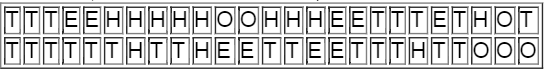×
Get Full Access to Elementary Statistics - 12 Edition - Chapter 2.2 - Problem 30bsc
Get Full Access to Elementary Statistics - 12 Edition - Chapter 2.2 - Problem 30bsc

×

# Categorical Data. In Exercise, use the given categoricalISBN: 9780321836960 18

## Solution for problem 30BSC Chapter 2.2

Elementary Statistics | 12th Edition

• Textbook Solutions
• 2901 Step-by-step solutions solved by professors and subject experts
• Get 24/7 help from StudySoup virtual teaching assistantsElementary Statistics | 12th Edition

4 5 1 280 Reviews
18
5
Problem 30BSC

Categorical Data. In Exercises 29–32, use the given categorical data to construct the relative frequency distribution.

Train Derailments An analysis of 50 train derailment incidents identified the main causes listed below, where T denotes bad track, E denotes faulty equipment, H denotes human error, and O denotes other causes (based on data from the Federal Railroad Administration).Step-by-Step Solution:

Step 1 of 1 :

Given, An analysis of 50 train derailment incidents identified the main causes listed below, where T denotes bad track, E denotes faulty equipment, H denotes human error, and O denotes other causes

That is

 Class Frequency Bad track (T) 23 Faulty equipment (E) 9 Human error (H) 12 Other causes (O) 6

We have to find the relative frequency

The total frequency = 50

Relative frequency =Example :

Relative frequency == 46

 Class Frequency Relative frequency Bad track (T) 23 46 Faulty equipment (E) 9 18 Human error (H) 12 24 Other causes (O) 6 12

Step 2 of 1

##### ISBN: 9780321836960

This full solution covers the following key subjects: denotes, data, categorical, train, causes. This expansive textbook survival guide covers 121 chapters, and 3629 solutions. Since the solution to 30BSC from 2.2 chapter was answered, more than 924 students have viewed the full step-by-step answer. The answer to “?Categorical Data. In Exercises 29–32, use the given categorical data to construct the relative frequency distribution.Train Derailments An analysis of 50 train derailment incidents identified the main causes listed below, where T denotes bad track, E denotes faulty equipment, H denotes human error, and O denotes other causes (based on data from the Federal Railroad Administration).” is broken down into a number of easy to follow steps, and 56 words. The full step-by-step solution to problem: 30BSC from chapter: 2.2 was answered by , our top Statistics solution expert on 03/15/17, 10:30PM. Elementary Statistics was written by and is associated to the ISBN: 9780321836960. This textbook survival guide was created for the textbook: Elementary Statistics, edition: 12.

Unlock Textbook Solution